# Electronics and Communication Engineering - Automatic Control Systems

### Exercise :: Automatic Control Systems - Section 2

26.

In a second order system, the time constant t of exponential envelopes depends

 A. only on damping factor B. only on natural frequency C. both on damping factor and natural frequency D. neither on damping factor nor on natural frequency

Explanation: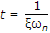27.

The maximum shift which can be provided by a lead compensator with transfer function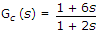is

 A. 15° B. 30° C. 45° D. 60°

Explanation:

The maximum phase lead is given by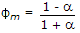In this case a = 1/3. Hence sin Φm =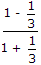= 0.5 or Φm = 30°.

28.

The network of the given figure is a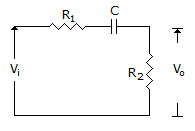A. lag network B. lead network C. lag-lead network D. any of the above

Explanation:

29.

For the given figure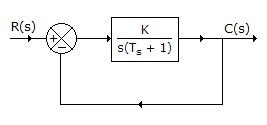A.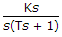B.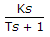C.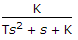D.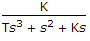Explanation: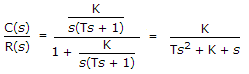.

30.

The resistance R of a liquid flow system is defined as

 A.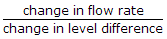B.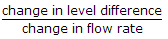C. change in level difference x change in flow rate D. non of the above

Electrical resistance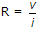, level difference or head is analogous to v and change in flow rate is analogous to current.